• 理论上发射和接收电磁波的...阵列天线的阵元数目、阵元间距、分布形式、激励相位和幅度5个因素决定了阵列天线波束方向的形成，决定天线的辐射特征。辐射方向可以描绘天线辐射特性随空间方向坐标变化关系。 阵列...
理论上发射和接收电磁波的任务可以由单个天线阵元构成的天线完成，但实际上天线要具有强方向性和高增益，要求天线波束可以扫描并具有一定形状，因此需要多个天线阵元构成阵列天线。阵列天线利用电磁波在空间相互干涉原理，构成不同形状阵列。 阵列天线的阵元数目、阵元间距、分布形式、激励相位和幅度5个因素决定了阵列天线波束方向图的形成，决定天线的辐射特征。辐射方向图可以描绘天线辐射特性随空间方向坐标变化关系。 阵列天线原理：叠加原理应用于阵列天线的远区辐射场。 假设一个阵列天线，由

M

M

个阵元组成，第

m

m

个阵元在阵中的方向图为

f

m

(

ϕ

,

θ

)

f_m(\phi,\theta)

，则整个阵列的方向图可以表示成

F

(

ϕ

,

θ

)

=

∑

m

=

1

M

f

m

(

ϕ

,

θ

)

S

m

F(\phi,\theta)=\sum^M_{m=1}f_m(\phi,\theta)S_m

若每个阵元的

f

m

(

ϕ

,

θ

)

f_m(\phi,\theta)

相同，则上式可以表示为

F

(

ϕ

,

θ

)

=

f

(

ϕ

,

θ

)

S

F(\phi,\theta)=f(\phi,\theta)S

式中

f

m

(

ϕ

,

θ

)

f_m(\phi,\theta)

也称为阵元因子，

S

S

表示为阵列因子，它和天线阵元在阵列中所处的位置有关。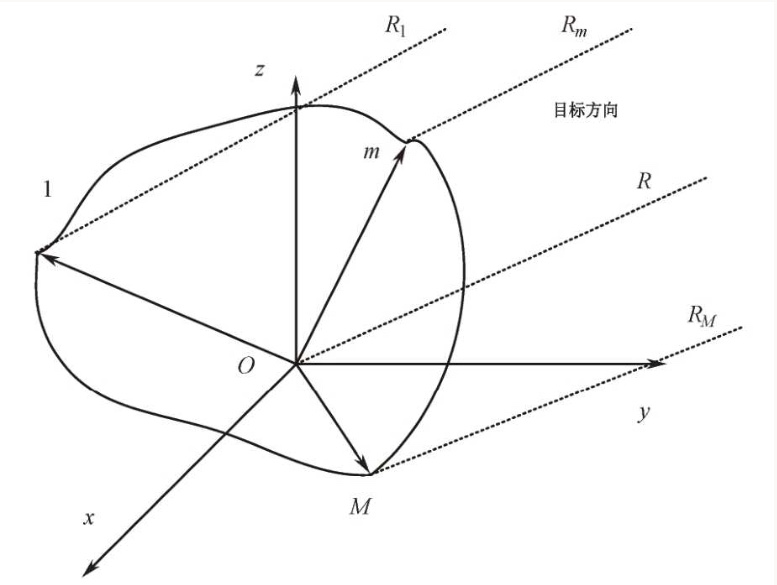设共有

M

M

个阵列天线阵元，第

m

m

个阵元在阵列中的位置为

(

x

m

,

y

m

,

z

m

)

(x_m,y_m,z_m)

，它的场强辐射方向图为

f

m

(

ϕ

,

θ

)

f_m(\phi,\theta)

，每个天线阵元的相位与幅度加权系数分别为

α

m

\alpha_m

和

A

m

A_m

，复加权系数

ω

m

\omega_m

可以表示为

ω

m

=

A

m

e

−

j

α

m

\omega_m=A_me^{-j\alpha_m}

则整个阵列所有天线阵元在

(

ϕ

,

θ

)

(\phi,\theta)

方向上的方向图可以表示为

F

(

ϕ

,

θ

)

=

∑

m

=

1

M

ω

m

⋅

f

m

(

ϕ

,

θ

)

e

−

j

2

π

λ

R

m

R

m

F(\phi,\theta)=\sum_{m=1}^M\omega_m·f_m(\phi,\theta)\frac{e^{-j\frac{2\pi}{\lambda}R_m}}{R_m}

R

m

=

R

−

Δ

R

m

R_m=R-\Delta R_m

，

Δ

R

m

\Delta R_m

是第

m

m

个天线阵元到目标的距离与参考点

O

O

到目标距离之间的差值，上式可以改写为

F

(

ϕ

,

θ

)

=

∑

m

=

1

M

f

m

(

ϕ

,

θ

)

A

m

e

j

(

2

π

λ

Δ

R

m

−

α

m

)

F(\phi,\theta)=\sum_{m=1}^Mf_m(\phi,\theta)A_me^{j(\frac{2\pi}{\lambda}\Delta R_m-\alpha_m)}

均匀直线阵列方向图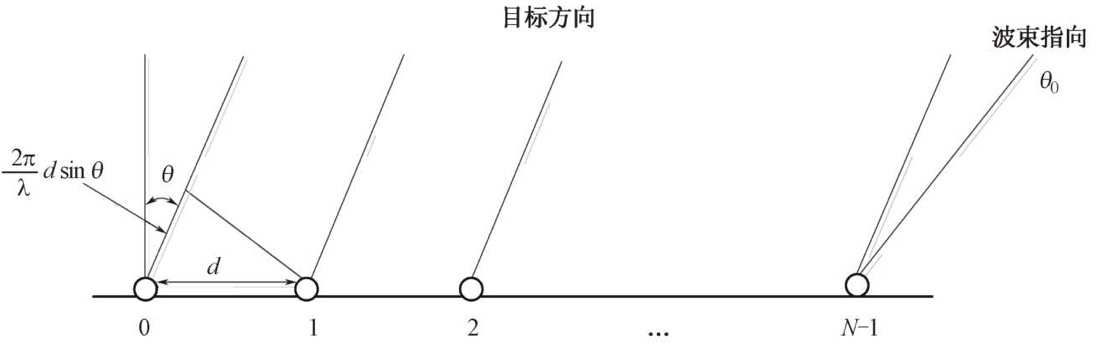假定天线阵元方向图

f

(

ϕ

,

θ

)

f(\phi,\theta)

足够宽，满足全向性，在线阵天线波束扫描范围内可忽略其影响，即

f

(

ϕ

,

θ

)

=

1

f(\phi,\theta)=1

；天线照射口径函数为等幅分布，即幅度加权系数

A

m

=

1

A_m=1

，满足均匀分布。线阵天线方向图函数可简化表示为

F

(

θ

)

=

∑

m

=

0

N

−

1

e

j

2

π

λ

d

m

(

sin

⁡

θ

−

sin

⁡

θ

0

)

F(\theta)=\sum_{m=0}^{N-1}e^{j\frac{2\pi}{\lambda}d_m(\sin\theta-\sin\theta_0)}

用MATLAB仿真： 设一直线阵列由20个阵元按半倍波长等间隔排列组成，所有阵元等幅全向，波长为1m，即阵列孔径为9.5m，天线波束指向为

0

∘

0^\circ

。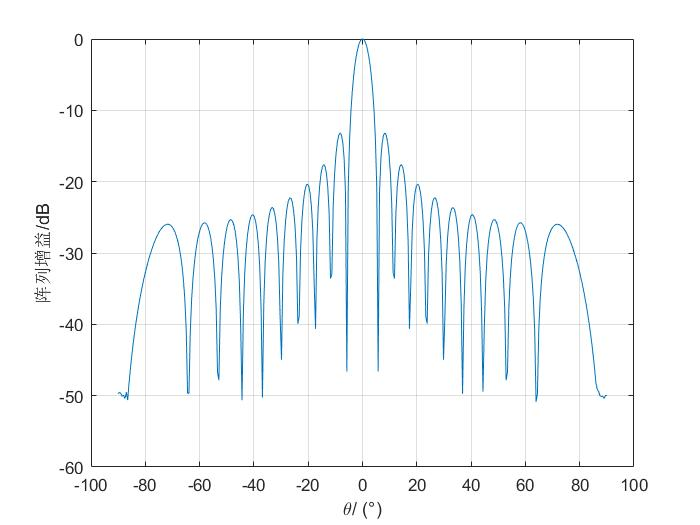均匀平面阵列方向图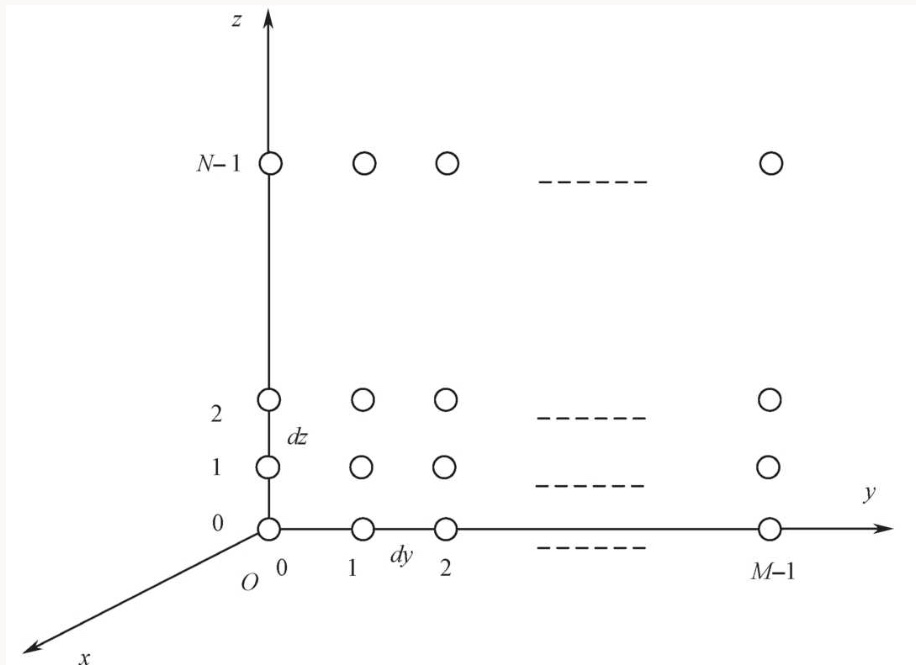天线阵列位于

y

o

z

yoz

平面上，共有

M

×

N

M×N

个天线阵元形成矩形栅格阵的平面阵列。平面阵列的方向图函数可以表示为：

F

(

ϕ

,

θ

)

=

∑

n

=

0

N

−

1

∑

m

=

0

M

−

1

f

m

n

(

ϕ

,

θ

)

A

m

n

e

j

(

Δ

ϕ

m

n

−

α

m

n

)

=

∑

n

=

0

N

−

1

∑

m

=

0

M

−

1

f

m

n

(

ϕ

,

θ

)

A

m

n

e

j

2

π

λ

[

d

m

(

cos

⁡

θ

sin

⁡

ϕ

−

cos

⁡

θ

0

sin

⁡

ϕ

0

)

+

d

n

(

sin

⁡

θ

−

sin

⁡

θ

0

)

]

\begin{aligned} F(\phi,\theta) &=\sum^{N-1}_{n=0}\sum_{m=0}^{M-1}f_{mn}(\phi,\theta)A_{mn}e^{j(\Delta \phi_{mn}-\alpha_{mn})}\\ &=\sum^{N-1}_{n=0}\sum_{m=0}^{M-1}f_{mn}(\phi,\theta)A_{mn}e^{j\frac{2\pi}{\lambda}[d_m(\cos\theta\sin\phi-\cos\theta_0\sin\phi_0)+d_n(\sin\theta-\sin\theta_0)]}\\ \end{aligned}

通常情况下，天线照射口径函数等幅分布，即幅度加权系数

A

m

n

=

1

A_{mn}=1

，满足均匀分布；假定天线阵列方向图

f

m

n

(

ϕ

,

θ

)

f_{mn}(\phi,\theta)

满足全向性，在线阵天线波束扫描范围内可忽略其影响，即

f

m

n

(

ϕ

,

θ

)

=

1

f_{mn}(\phi,\theta)=1

，则平面阵列天线的方向图函数可表示为

F

(

ϕ

,

θ

)

=

∑

n

=

0

N

−

1

∑

m

=

0

M

−

1

e

j

2

π

λ

[

d

m

(

cos

⁡

θ

sin

⁡

ϕ

−

cos

⁡

θ

0

sin

⁡

ϕ

0

)

+

d

n

(

sin

⁡

θ

−

sin

⁡

θ

0

)

]

=

∑

m

=

0

M

−

1

e

j

2

π

λ

d

m

(

cos

⁡

θ

sin

⁡

ϕ

−

cos

⁡

θ

0

sin

⁡

ϕ

0

)

∑

n

=

0

N

−

1

e

j

2

π

λ

d

n

(

sin

⁡

θ

−

sin

⁡

θ

0

)

=

∣

F

1

(

ϕ

,

θ

)

∣

⋅

∣

F

2

(

θ

)

∣

\begin{aligned} F(\phi,\theta) &=\sum^{N-1}_{n=0}\sum_{m=0}^{M-1}e^{j\frac{2\pi}{\lambda}[d_m(\cos\theta\sin\phi-\cos\theta_0\sin\phi_0)+d_n(\sin\theta-\sin\theta_0)]}\\ &=\sum_{m=0}^{M-1}e^{j\frac{2\pi}{\lambda}d_m(\cos\theta\sin\phi-\cos\theta_0\sin\phi_0)}\sum^{N-1}_{n=0}e^{j\frac{2\pi}{\lambda}d_n(\sin\theta-\sin\theta_0)}\\ &=|F_1(\phi,\theta)|·|F_2(\theta)| \end{aligned}

∣

F

1

(

ϕ

,

θ

)

∣

|F_1(\phi,\theta)|

表示水平方向线阵的天线方向图，

∣

F

2

(

θ

)

∣

|F_2(\theta)|

表示垂直方向线阵的天线方向图。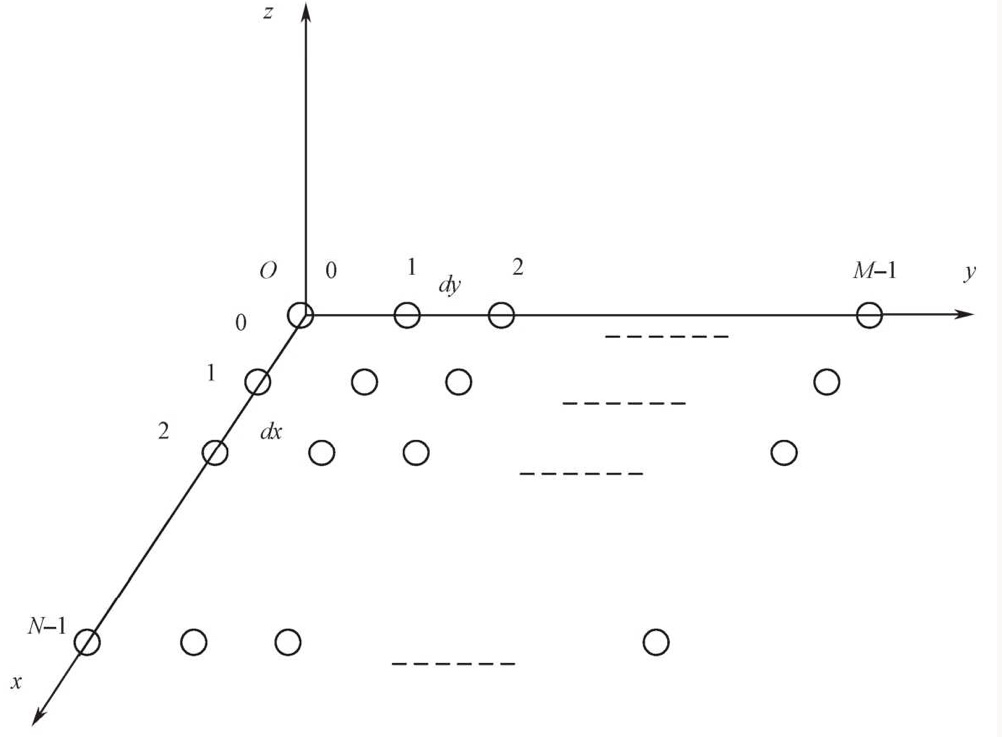天线阵列位于

x

o

y

xoy

平面上，共有

M

×

N

M×N

个天线阵元形成矩形栅格阵的平面阵列。平面阵列的方向图函数可以表示为：

F

(

ϕ

,

θ

)

=

∑

n

=

0

N

−

1

∑

m

=

0

M

−

1

e

j

2

π

λ

[

d

n

(

sin

⁡

θ

cos

⁡

ϕ

−

sin

⁡

θ

0

cos

⁡

ϕ

0

)

+

d

m

(

sin

⁡

θ

sin

⁡

ϕ

−

sin

⁡

θ

0

sin

⁡

ϕ

0

)

]

=

∑

m

=

0

M

−

1

e

j

2

π

λ

d

m

(

sin

⁡

θ

sin

⁡

ϕ

−

sin

⁡

θ

0

sin

⁡

ϕ

0

)

∑

n

=

0

N

−

1

e

j

2

π

λ

d

n

(

sin

⁡

θ

cos

⁡

ϕ

−

sin

⁡

θ

0

cos

⁡

ϕ

0

)

=

∣

F

1

(

ϕ

,

θ

)

∣

⋅

∣

F

2

(

ϕ

,

θ

)

∣

\begin{aligned} F(\phi,\theta) &=\sum^{N-1}_{n=0}\sum_{m=0}^{M-1}e^{j\frac{2\pi}{\lambda}[d_n(\sin\theta\cos\phi-\sin\theta_0\cos\phi_0)+d_m(\sin\theta\sin\phi-\sin\theta_0\sin\phi_0)]}\\ &=\sum_{m=0}^{M-1}e^{j\frac{2\pi}{\lambda}d_m(\sin\theta\sin\phi-\sin\theta_0\sin\phi_0)}\sum^{N-1}_{n=0}e^{j\frac{2\pi}{\lambda}d_n(\sin\theta\cos\phi-\sin\theta_0\cos\phi_0)}\\ &=|F_1(\phi,\theta)|·|F_2(\phi,\theta)| \end{aligned}

∣

F

1

(

ϕ

,

θ

)

∣

|F_1(\phi,\theta)|

表示

y

y

方向线阵的天线方向图，

∣

F

2

(

ϕ

,

θ

)

∣

|F_2(\phi,\theta)|

表示

x

x

方向线阵的天线方向图。
用MATLAB仿真： 设一均匀平面阵列由20行方位向阵元和10列俯仰向阵元按半倍波长等间隔排列组成，所有阵元等幅全向，波长为1m，即阵列孔径为9.5m×4.5m，天线波束指向为

(

0

∘

,

0

∘

)

(0^\circ,0^\circ)

。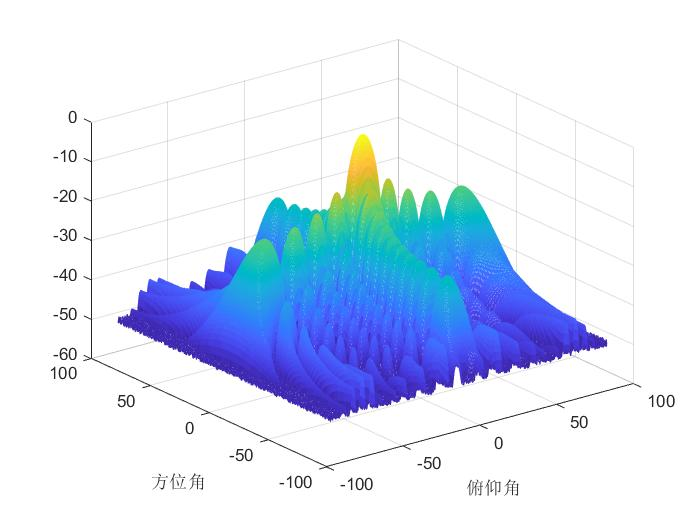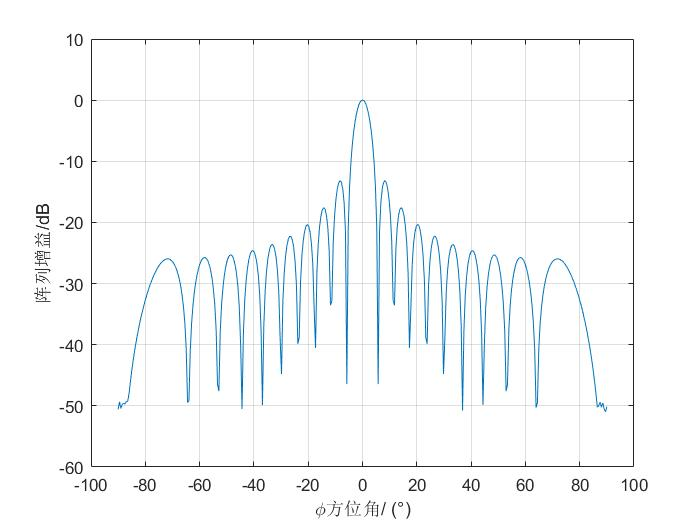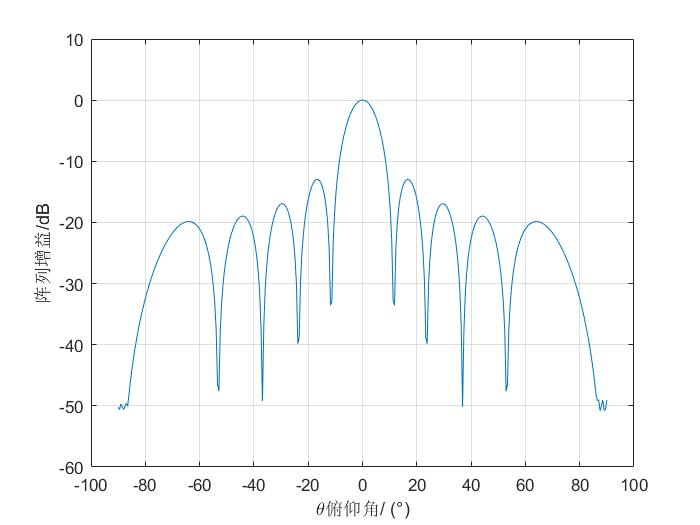展开全文• 自己在学习半平面交的时候看到网上有几张图片很不错就 down 下来在本文中使用了……如果博主大大不乐意请联系我删除。下面给出两个我觉得不错的博客： 1.半平面交讲解1 2.半平面交讲解2 定义： 半平面：...
欢迎关注我的个人博客：www.zuzhiang.cn

自己在学习半平面交的时候看到网上有几张图片很不错就 down 下来在本文中使用了……如果博主大大不乐意请联系我删除。下面给出两个我觉得不错的博客：
1.半平面交讲解1
2.半平面交讲解2

定义：
半平面：顾名思义，就是平面的一半。一条直线会把平面分成两部分，就是两个半平面。对于半平面，我们可以用直线方程式如：ax + by >= c 表示，更常用的是用向量表示，默认向量的左侧为我们所需要的半平面。

半平面交：顾名思义，就是多个半平面求交集。其结果可能是一个凸多边形、无穷平面、直线、线段、点等。

多边形的核：如果多边形中存在一个区域使得在区域中可以看到多边形中任意位置（反之亦然），则这个区域就是多边形的核。可以用半平面交来求解。

极点：听说过极坐标吧？极点就是点 (x,y) 与原点的连线与 x 轴的夹角，其范围为 [0,360].

应用：
1.判断多边形的核是否存在。
2.求多边形中可以放入的最大圆半径。

代码：
下面给出两种不同风格的代码，一种是网上找的，便于理解，一种是从kuangbin模板上摘下来的，适用性更广，可以当作板子用。其中对于给出点的顺时针和逆时针顺序不同，邝斌模板只需要加个 reverse 函数将点的顺序颠倒，而第一个模板需要更改 cmp函数和 Judge函数中的 < 或 >。

int n,tol,bot,top; //bot和top指向双端队列的底部和顶部
int dq[MAXN],order[MAXN]; //双端队列和存储排序后点的下标

struct point
{ //点
double x,y;
} p[MAXN];

struct vct
{ //向量（直线）
point start,end;
double angle; //极角
} v[MAXN],tmp[MAXN];

int dbcmp(double k)
{ //判断为0，还是为正负
if(fabs(k)<eps) return 0;
return k>0? 1:-1;
}

double Multi(point p0,point p1,point p2)
{ //向量 p0p1 和 p0p2 相乘
return (p1.x-p0.x)*(p2.y-p0.y)-(p2.x-p0.x)*(p1.y-p0.y);
}

int cmp(int x,int y)
{ //排序的比较函数
int d=dbcmp(v[x].angle-v[y].angle);
if(!d) return dbcmp(Multi(v[x].start,v[y].start,v[y].end))>0;
//大于0取向量左半部分为半平面，小于0，取右半部分
return d<0;
}

void addline(double x1,double y1,double x2,double y2)
{ //增加一个向量（直线）
v[tol].start.x=x1;
v[tol].start.y=y1;
v[tol].end.x=x2;
v[tol].end.y=y2;
//atan2是求原点到(x,y)的直线与x轴的夹角，比atan稳定
v[tol].angle=atan2(y2-y1,x2-x1);
//order[tol]=tol;
tol++;
}

void GetIntersect(vct v1,vct v2,point &p)
{ //获取交点并返回给 p
double dot1,dot2;
dot1=Multi(v1.start,v2.end,v1.end);
dot2=Multi(v1.start,v2.start,v1.end);
p.x=(dot1*v2.start.x-dot2*v2.end.x)/(dot1-dot2);
p.y=(dot1*v2.start.y-dot2*v2.end.y)/(dot1-dot2);
}

int Judge(vct v0,vct v1,vct v2)
{ //判断 v1 和 v2 的交点 pt 在半平面 v0内
point pt;
GetIntersect(v1,v2,pt);
return dbcmp(Multi(pt,v0.start,v0.end))<0;
//大于0 pt在 v0 的左面，小于0 pt 在 v0 的右面
//如果 pt 在半平面 v0 内则返回真
}

int HalfPlaneIntersection()
{ //求解半平面交
int i,j;
for(i=0;i<tol;i++) order[i]=i;
sort(order,order+n,cmp); //极角排序
for(i=1,j=0;i<tol;i++) //排除极角相同的
if(dbcmp(tmp[order[i]].angle-tmp[order[j]].angle)>0)
order[++j]=order[i];
n=j+1; //个数
dq=order; //开始时入队两条直线
dq=order;
bot=0;
top=1;
for(i=2;i<tol;i++)
{ //如果双端队列底端或顶端两个半平面的交点在当前半平面之外，则删除
while(bot<top&&Judge(tmp[order[i]],tmp[dq[top-1]],tmp[dq[top]]))
top--;
while(bot<top&&Judge(tmp[order[i]],tmp[dq[bot+1]],tmp[dq[bot]]))
bot++;
dq[++top]=order[i]; //将当前半平面加入队列的顶端
}
while(bot<top&&Judge(tmp[dq[bot]],tmp[dq[top-1]],tmp[dq[top]])) top--;
while(bot<top&&Judge(tmp[dq[top]],tmp[dq[bot+1]],tmp[dq[bot]])) bot++;
if(top-bot<=1) return 0;
else return 1;
}

double GetDis(point a,point b)
{
return sqrt((a.x-b.x)*(a.x-b.x)+(a.y-b.y)*(a.y-b.y));
}

void ChangePolygon(double h)
{ //将所有边往内缩进 h距离
int i;
double dx,dy,len;
for(i=0;i<tol;i++)
{
len=GetDis(v[i].start,v[i].end);
dx=(v[i].start.y-v[i].end.y)/len*h;
dy=(v[i].end.x-v[i].start.x)/len*h;
tmp[i].start.x=v[i].start.x+dx;
tmp[i].start.y=v[i].start.y+dy;
tmp[i].end.x=v[i].end.x+dx;
tmp[i].end.y=v[i].end.y+dy;
tmp[i].angle=v[i].angle;
}
}

double BSearch()
{ //二分搜索
double l=0,r=20000,mid;
while(l+eps<r)
{
mid=(l+r)/2;
ChangePolygon(mid); //将所有边往内缩进 mid距离
if(HalfPlaneIntersection()) l=mid; //如果存在半平面交
else r=mid;
}
return l;
}

int sgn(double x)
{ //符号函数
if(fabs(x) < eps) return 0;
if(x < 0) return -1;
else return 1;
}

struct Point
{ //点
double x,y;
Point(){}
Point(double _x,double _y)
{
x = _x; y = _y;
}
Point operator -(const Point &b)const
{
return Point(x - b.x, y - b.y);
}
double operator ^(const Point &b)const
{ //叉积
return x*b.y - y*b.x;
}
double operator *(const Point &b)const
{ //点积
return x*b.x + y*b.y;
}
};

struct Line
{ //向量
Point s,e; //两点
double k; //斜率
Line(){}
Line(Point _s,Point _e)
{ //构造
s = _s; e = _e;
k = atan2(e.y - s.y,e.x - s.x);
}
Point operator &(const Line &b)const
{ //求两直线交点
Point res = s;
double t = ((s - b.s)^(b.s - b.e))/((s - e)^(b.s - b.e));
res.x += (e.x - s.x)*t;
res.y += (e.y - s.y)*t;
return res;
}
};

Line Q[MAXN];
Point p[MAXN]; //记录最初给的点集
Line line[MAXN]; //由最初的点集生成直线的集合
Point pp[MAXN]; //记录半平面交的结果的点集

//半平面交，直线的左边代表有效区域
bool HPIcmp(Line a,Line b)
{ //直线排序函数
if(fabs(a.k - b.k) > eps)return a.k < b.k; //斜率排序
//斜率相同我也不知道怎么办
return ((a.s - b.s)^(b.e - b.s)) < 0;
}

void HPI(Line line[], int n, Point res[], int &resn)
{ //line是半平面交的直线的集合 n是直线的条数 res是结果
//的点集 resn是点集里面点的个数
int tot = n;
sort(line,line+n,HPIcmp);
tot = 1;
for(int i = 1;i < n;i++)
if(fabs(line[i].k - line[i-1].k) > eps) //去掉斜率重复的
line[tot++] = line[i];
int head = 0, tail = 1;
Q = line;
Q = line;
resn = 0;
for(int i = 2; i < tot; i++)
{
if(fabs((Q[tail].e-Q[tail].s)^(Q[tail-1].e-Q[tail-1].s)) < eps ||
return;
while(head < tail && (((Q[tail]&Q[tail-1]) -
line[i].s)^(line[i].e-line[i].s)) > eps)
tail--;
line[i].s)^(line[i].e-line[i].s)) > eps)
Q[++tail] = line[i];
}
while(head < tail && (((Q[tail]&Q[tail-1]) -
tail--;
Q[tail].s)^(Q[tail].e-Q[tail].e)) > eps)
if(tail <= head + 1) return;
for(int i = head; i < tail; i++)
res[resn++] = Q[i]&Q[i+1];
}

double dist(Point a,Point b)
{ //两点间距离
return sqrt((a-b)*(a-b));
}

void change(Point a,Point b,Point &c,Point &d,double p)
{ //将线段ab往左移动距离p,修改得到线段cd
double len=dist(a,b);
/*三角形相似推出下面公式*/
double dx=(a.y-b.y)*p/len;
double dy=(b.x-a.x)*p/len;
c.x=a.x+dx; c.y=a.y+dy;
d.x=b.x+dx; d.y=b.y+dy;
}

double BSearch()
{ //二分搜索
double l=0,r=100000;
double ans=0;
while(r-l>=eps)
{
double mid=(l+r)/2;
for(int i=0;i < n;i++)
{
Point t1,t2;
change(p[i],p[(i+1)%n],t1,t2,mid);
line[i]=Line(t1,t2);
}
int resn;
HPI(line,n,pp,resn);
//等于0说明移多了
if(resn==0) r=mid-eps;
else l=mid+eps;
}
return l;
}

半平面交算法：
最基本的是时间复杂度为 O(n) 的算法，常用的是时间复杂度为 O(nlogn) 的排序增量算法。

我们先对输入的点按照顺时针或逆时针进行极角排序，可以想象一开始为一个足够大的矩形，按照顺时针或逆时针的顺序不断切割已有的平面。求 n 个半平面的交就是用第 n 条表示当前半平面的直线去切割现有的面。每次切割都会产生一个更小的面，当所有直线都切割完毕后就是我们所需要的结果了。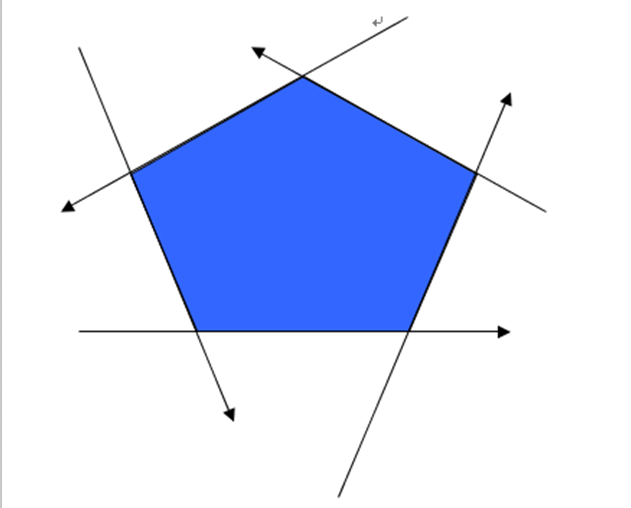排序时，我们将向量平移至以原点为起点，将坐标轴以 x 轴为界分为上下两部（x 轴属于下半部分），当两个向量终点的 y 都在 x 轴上时，按 x 从小到大排序。当两个向量重点同在上部/下部时，按叉积排序（平行按左右排序），当一上一下时，下部的排在前。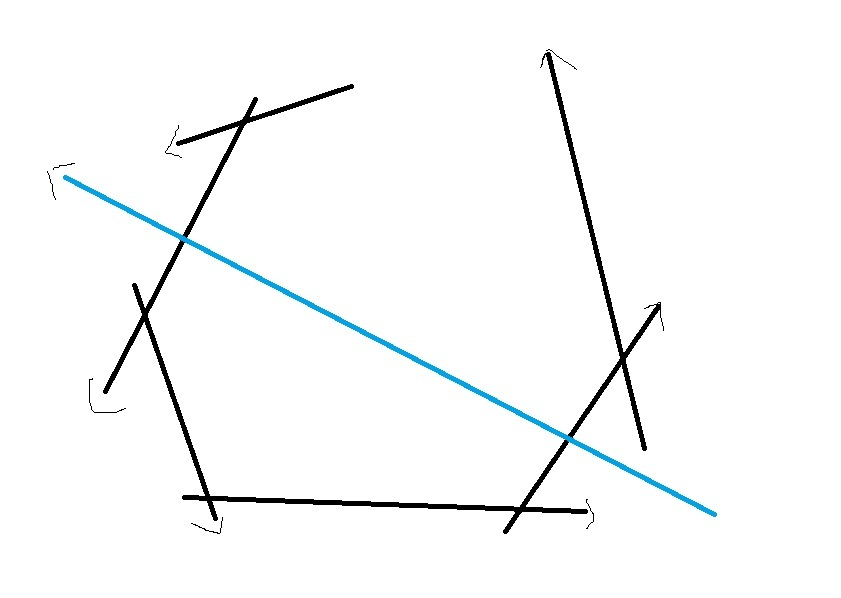在切割的时候我们可以用一个双端队列来保存属于半平面交内的点。如上图所示，当用一条新的直线切割已有平面的时候，可能不在半平面交内的点只可能是两端的点，所以每次就不用全部点判断一次，只需要判断双端队列两端的点就可以了。

多边形的核求解方法：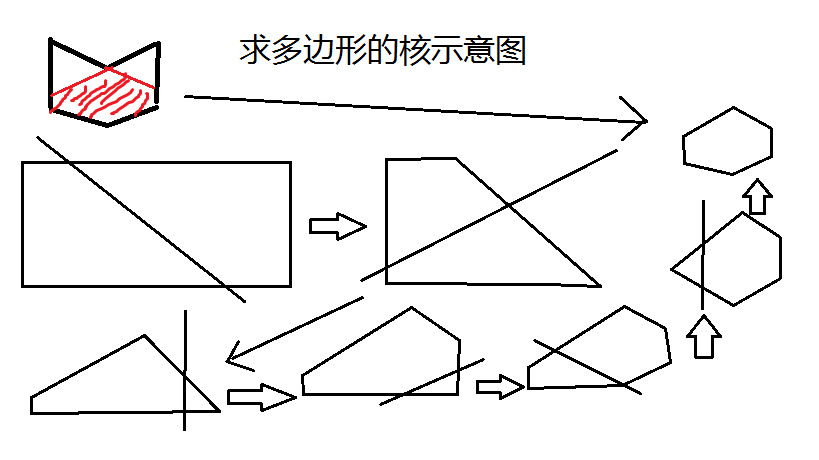前面说过判断多边形是否存在核可以用半平面交来解决。如上图所示，左上角是要求的多边形。我们可以按照顺时针的顺序用多边形的边切割一个足够大的矩形，最终剩下的就是多边形的核。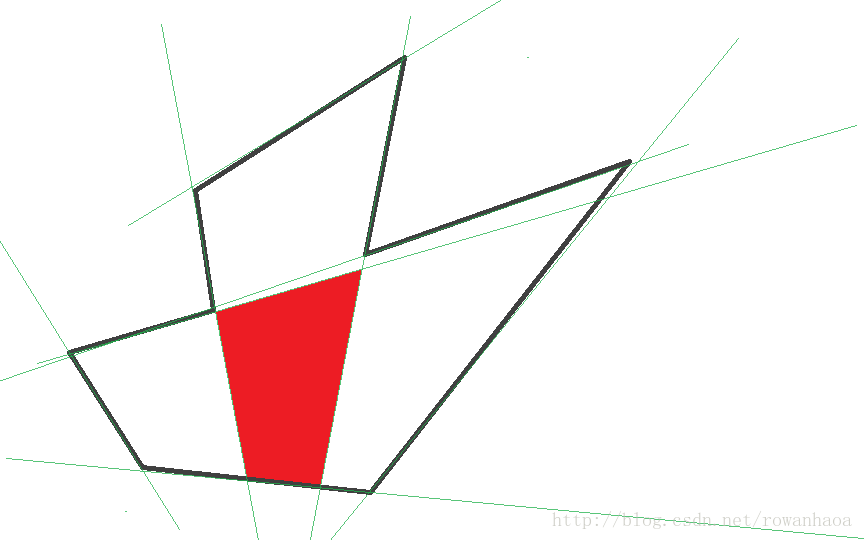求多边形中能够放入的最大圆的半径：
可以放入的最大圆的圆心（不是圆本身）一定在多边形的核内，别问我为什么，鬼才知道……我们可以假设，最大圆半径为 r 。如果我们可以将多边形的每条边向内推进 r 长度的距离，这时候可以想见多边形里面放不开这个圆了，并且圆心所在的多边形的核也一定是变成了一个点。否则就多边形的边就还可以向内推进，这个圆就不是最大的了。
当我们不知道最大圆半径的时候，我们可以用二分的方法求半径的值，如果对于当前假设的圆半径 r ，每条边向内推进 r 距离后，发现多边形的核不存在了，则说明当前圆半径太大了，反之，就是圆半径小了，用二分找到最大的圆半径即可。

例题：
1.POJ 3335
2.POJ 3130
题意：其问题都是判断多边形的核是否存在，具体思路当然是用半平面交来解决了。要注意的是两个题给出的点的数序不同，一个是顺时针一个是逆时针，所以代码中有些地方也会有所差异。
题解：POJ 3130&3335题解。

3.POJ 3525
题意：求多边形内到边上距离最远的距离。可以转化为求多边形内可以放入的最大的圆的半径。
题解：POJ 3525 题解。

4.POJ 3384
题意：将两个半径为 r 的圆放入一个多边形中，两圆可以重叠，问两个圆占据的最大面积时的圆心坐标是多少，可以转化为上一题的方法解决。
题解：POJ 3384 题解。

展开全文多边形的核 计算几何
• 平面（hyperplane）的定义： {x∣aTx=b}{x∣aTx=b}\{x\mid a^{T}x=b\} 其中 aaa 是一个非零向量，bbb 是实数，即 a≠0,b∈Ra≠0,b∈Ra\neq 0, b\in R. 几何意义是：从满足条件 ax0=bax0=bax_0=b 的任意一点 x0...
它们都是凸集
超平面（hyperplane）的定义：

{

x

∣

a

T

x

=

b

}

\{x\mid a^{T}x=b\}

其中

a

a

是一个非零向量，

b

b

是实数，即

a

≠

0

,

b

∈

R

a\neq 0, b\in R

. 即一个方程。 几何意义是：从满足条件

a

T

x

0

=

b

a^Tx_0=b

的任意一点

x

0

x_0

出发的向量

a

a

的所有垂线的集合。 例如下图中的黑体箭头（图比较难画，直接粘贴书上的了）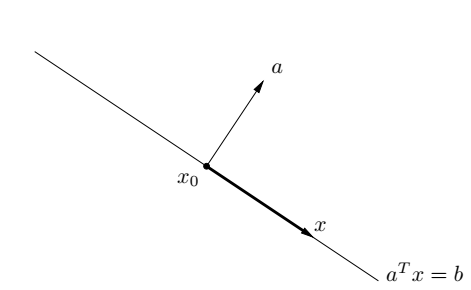一个超平面有两个半空间，半空间定义：

{

x

∣

a

T

x

≤

b

}

\{x\mid a^{T}x\leq b\}

多面体定义（Polyhedron）：

P

=

{

x

∣

A

x

≺

b

,

C

x

=

d

}

\mathcal{P}=\{x\mid Ax\prec b, Cx=d\}

它表示有限个半空间和超平面的交集。
多面体是一个凸集，单纯形（三角形，四面体）也是一个多面体
展开全文• ## Matlab绘图

千次阅读 2018-05-27 10:36:24
平面网格坐标矩阵的生成 当绘制z=f(x,y)所代表的三维曲面时，先要在xy平面选定一矩形区域，假定矩形区域为D＝[a,b]×[c,d]，然后将[a,b]在x方向分成m份，将[c,d]在y方向分成n份，由各划分点做平行轴的直线，把区域...

Matlab绘图
强大的绘图功能是Matlab的特点之一，Matlab提供了一系列的绘图函数，用户不需要过多的考虑绘图的细节，只需要给出一些基本参数就能得到所需图形，这类函数称为高层绘图函数。此外，Matlab还提供了直接对图形句柄进行操作的低层绘图操作。这类操作将图形的每个图形元素（如坐标轴、曲线、文字等）看做一个独立的对象，系统给每个对象分配一个句柄，可以通过句柄对该图形元素进行操作，而不影响其他部分。
本章介绍绘制二维和三维图形的高层绘图函数以及其他图形控制函数的使用方法，在此基础上，再介绍可以操作和控制各种图形对象的低层绘图操作。
一．二维绘图
二维图形是将平面坐标上的数据点连接起来的平面图形。可以采用不同的坐标系，如直角坐标、对数坐标、极坐标等。二维图形的绘制是其他绘图操作的基础。
一．绘制二维曲线的基本函数
在Matlab中，最基本而且应用最为广泛的绘图函数为plot，利用它可以在二维平面上绘制出不同的曲线。
1． plot函数的基本用法
plot函数用于绘制二维平面上的线性坐标曲线图，要提供一组x坐标和对应的y坐标，可以绘制分别以x和y为横、纵坐标的二维曲线。plot函数的应用格式
plot(x,y)     其中x,y为长度相同的向量，存储x坐标和y坐标。
例51 在[0 , 2pi]区间，绘制曲线
程序如下：在命令窗口中输入以下命令
>> x=0:pi/100:2*pi;
>> y=2*exp(-0.5*x).*sin(2*pi*x);
>> plot(x,y)
程序执行后，打开一个图形窗口，在其中绘制出如下曲线
注意：指数函数和正弦函数之间要用点乘运算，因为二者是向量。

例52 绘制曲线
这是以参数形式给出的曲线方程，只要给定参数向量，再分别求出x,y向量即可输出曲线：
>> t=-pi:pi/100:pi;
>> x=t.*cos(3*t);
>> y=t.*sin(t).*sin(t);
>> plot(x,y)
程序执行后，打开一个图形窗口，在其中绘制出如下曲线

以上提到plot函数的自变量x,y为长度相同的向量，这是最常见、最基本的用法。实际应用中还有一些变化。分别说明：
①
2． 含多个输入参数的plot函数
plot函数可以包含若干组向量对，每一组可以绘制出一条曲线。含多个输入参数的plot函数调用格式为：plot(x1，y1，x2，y2，…，xn，yn)
如下列命令可以在同一坐标中画出3条曲线。
>> x=linspace(0,2*pi,100);
>> plot(x,sin(x),x,2*sin(x),x,3*sin(x))

当输入参数有矩阵形式时，配对的x,y按对应的列元素为横坐标和纵坐标绘制曲线，曲线条数等于矩阵的列数。

>> x=linspace(0,2*pi,100);
>> y1=sin(x);
>> y2=2*sin(x);
>> y3=3*sin(x);
>> x=[x;x;x]';
>> y=[y1;y2;y3]';
>> plot(x,y,x,cos(x))
x,y都是含有三列的矩阵，它们组成输入参数对，绘制三条曲线；x和cos(x)又组成一对，绘制一条余弦曲线。
利用plot函数可以直接将矩阵的数据绘制在图形窗体中，此时plot函数将矩阵的每一列数据作为一条曲线绘制在窗体中。如
>> A=pascal(5)
A =
1     1     1     1     1
1     2     3     4     5
1      3     6    10    15
1     4    10    20    35
1     5    15    35    70
>> plot(A)

3． 含选项的plot函数
Matlab提供了一些绘图选项，用于确定所绘曲线的线型、颜色和数据点标记符号。这些选项如表所示：
线型  颜色  标记符号  - 实线  b蓝色  .   点  s 方块  : 虚线  g绿色  o 圆圈  d 菱形  -. 点划线  r红色  × 叉号  ∨朝下三角符号  -- 双划线  c青色  + 加号  ∧朝上三角符号     m品红  * 星号  <朝左三角符号     y黄色     >朝右三角符号     k黑色     p 五角星     w白色     h 六角星

例 用不同的线型和颜色在同一坐标内绘制曲线 及其包络线。
>> x=(0:pi/100:2*pi)';
>> y1=2*exp(-0.5*x)*[1,-1];
>> y2=2*exp(-0.5*x).*sin(2*pi*x);
>> x1=(0:12)/2;
>> y3=2*exp(-0.5*x1).*sin(2*pi*x1);
>> plot(x,y1,'k:',x,y2,'b--',x1,y3,'rp');

在该plot函数中包含了3组绘图参数，第一组用黑色虚线画出两条包络线，第二组用蓝色双划线画出曲线y，第三组用红色五角星离散标出数据点。
4． 双纵坐标函数plotyy
在Matlab中，如果需要绘制出具有不同纵坐标标度的两个图形，可以使用plotyy函数，它能把具有不同量纲，不同数量级的两个函数绘制在同一个坐标中，有利于图形数据的对比分析。使用格式为：plotyy(x1,y1,x2,y2)
x1,y1对应一条曲线，x2,y2对应另一条曲线。横坐标的标度相同，纵坐标有两个，左边的对应x1,y1数据对，右边的对应x2,y2。
例：（略）
二．绘制图形的辅助操作
绘制完图形以后，可能还需要对图形进行一些辅助操作，以使图形意义更加明确，可读性更强。
1． 图形标注
在绘制图形时，可以对图形加上一些说明，如图形的名称、坐标轴说明以及图形某一部分的含义等，这些操作称为添加图形标注。有关图形标注函数的调用格式为：
title（’图形名称’） （都放在单引号内）
xlabel（’x轴说明’）
ylabel（’y轴说明’）
text（x，y，’图形说明’）
legend（’图例1’，’图例2’，…） P190
其中，title、xlabel和ylabel函数分别用于说明图形和坐标轴的名称。text函数是在坐标点（x，y）处添加图形说明。（P88 或用gtext命令）。legend函数用于绘制曲线所用线型、颜色或数据点标记图例，图例放置在空白处，用户还可以通过鼠标移动图例，将其放到所希望的位置。除legend函数外，其他函数同样适用于三维图形，在三维中z坐标轴说明用zlabel函数。
上述函数中的说明文字，除了使用标准的ASCII字符外，还可以使用LaTex（一种流行的数学排版软件）格式的控制字符，这样就可以在图形上添加希腊字符，数学符号和公式等内容。在Matlab支持的LaTex字符串中，用/bf , /it , /rm控制字符分别定义黑体、斜体和正体字符，受LaTex字符串控制部分要加大括号{}括起来。例如，text(0.3，0.5，’the usful {/bf MATLAB}’)，将使MATLAB一词黑体显示。一些常用的LaTex字符见表，各个字符可以单独使用也可以和其他字符及命令配合使用。如text(0.3 ,0.5 ,’sin({/omega}t+{/beta})’)
将得到标注效果 。
标识符  符号  标识符  符号  标识符  符号  /alpha     /epsilon     /infty     /beta     /eta     /int     /gamma     /Gamma     /partial     /delta     /Delta     /leftarrow     /theta     /Theta     /rightarrow     /lambda     /Lambda     /downarrow     /xi     /Xi     /uparrow     /pi     /Pi     /div     /omega     /Omega     /times     /sigma     /Sigma     /pm     /phi     /Phi     /leq     /psi     /Psi     /geq     /rho     /tau     /neq     /mu     /zeta     /forall     /nu     /chi     /exists
2． 坐标控制
在绘制图形时，Matlab可以自动根据要绘制曲线数据的范围选择合适的坐标刻度，使得曲线能够尽可能清晰的显示出来。所以，一般情况下用户不必选择坐标轴的刻度范围。但是，如果用户对坐标不满意，可以利用axis函数对其重新设定。其调用格式为
axis（[xmin xmax ymin ymax zmin zmax]）
如果只给出前四个参数，则按照给出的x、y轴的最小值和最大值选择坐标系范围，绘制出合适的二维曲线。如果给出了全部参数，则绘制出三维图形。
axis函数的功能丰富，其常用的用法有：
axis equal ：纵横坐标轴采用等长刻度
axis square：产生正方形坐标系（默认为矩形）
axis auto：使用默认设置
axis off：取消坐标轴
axis on ：显示坐标轴
还有：给坐标加网格线可以用grid命令来控制，grid on/off命令控制画还是不画网格线，不带参数的grid命令在两种之间进行切换。
给坐标加边框用box命令控制。和grid一样用法
例 ：绘制分段函数，并添加图形标注。（略）
3． 图形保持
一般情况下，每执行一次绘图命令，就刷新一次当前图形窗口，图形窗口原有图形将不复存在，如果希望在已经存在的图形上再继续添加新的图形，可以使用图形保持命令hold。hold on/off 命令是保持原有图形还是刷新原有图形，不带参数的hold命令在两者之间进行切换。
例：（略）
4． 图形窗口分割
在实际应用中，经常需要在一个图形窗口中绘制若干个独立的图形，这就需要对图形窗口进行分割。分割后的图形窗口由若干个绘图区组成，每一个绘图区可以建立独立的坐标系并绘制图形。同一图形窗口下的不同图形称为子图。Matlab提供了subplot函数用来将当前窗口分割成若干个绘图区，每个区域代表一个独立的子图，也是一个独立的坐标系，可以通过subplot函数激活某一区，该区为活动区，所发出的绘图命令都是作用于该活动区域。调用格式：
subplot（m，n，p）
该函数把当前窗口分成m×n个绘图区，m行，每行n个绘图区，区号按行优先编号。其中第p个区为当前活动区。每一个绘图区允许以不同的坐标系单独绘制图形。
例：（略）
三．绘制二维图形的其他函数
1． 其他形式的线性直角坐标图
在线性直角坐标中，其他形式的图形有条形图、阶梯图、杆图和填充图等，所采用的函数分别为：
bar（x，y，选项）      选项在单引号中
stairs（x，y，选项）
stem（x，y，选项）
fill（x1，y1，选项1，x2，y2，选项2，…）
前三个函数和plot的用法相似，只是没有多输入变量形式。fill函数按向量元素下标渐增次序依次用直线段连接x，y对应元素定义的数据点。
例5-8：分别以条形图、填充图、阶梯图和杆图形式绘制曲线
x=0:0.35:7;
y=2*exp(-0.5*x);
subplot(2,2,1);bar(x,y,'g');
title('bar(x,y,''g'')');axis([0, 7, 0 ,2]);
subplot(2,2,2);fill(x,y,'r');
title('fill(x,y,''r'')');axis([0, 7, 0 ,2]);
subplot(2,2,3);stairs(x,y,'b');
title('stairs(x,y,''b'')');axis([0, 7, 0 ,2]);
subplot(2,2,4);stem(x,y,'k');
title('stem(x,y,''k'')');axis([0, 7, 0 ,2]);

2． 极坐标图
polar函数用来绘制极坐标图，调用格式为：
polar（theta，rho，选项）
其中，theta为极坐标极角，rho为极径，选项的内容和plot函数相似。
例5-9：绘制 的极坐标图

theta=0:0.01:2*pi;
rho=sin(3*theta).*cos(5*theta);
polar(theta,rho,'r');
3． 对数坐标图
在实际应用中，经常用到对数坐标，Matlab提供了绘制对数和半对数坐标曲线的函数，其调用格式为：
semilogx（x1，y1，选项1，x2，y2，选项2，…）
semilogy（x1，y1，选项1，x2，y2，选项2，…）
loglog（x1，y1，选项1，x2，y2，选项2，…）
这些函数中选项的定义和plot函数完全一样，所不同的是坐标轴的选取。semilogx函数使用半对数坐标，x轴为常用对数刻度，而y轴仍保持线性刻度。semilogy恰好和semilogx相反。loglog函数使用全对数坐标，x、y轴均采用对数刻度。
例：略
4． 对函数自适应采样的绘图函数
5． 其他形式的二维图形
二． 三维绘图
一．绘制三维曲线的基本函数
最基本的三维图形函数为plot3，它将二维绘图函数plot的有关功能扩展到三维空间，可以用来绘制三维曲线。其调用格式为：
plot3（x1，y1，z1，选项1，x2，y2，z2，选项2，…）
其中每一组x，y，z组成一组曲线的坐标参数，选项的定义和plot的选项一样。当x，y，z是同维向量时，则x，y，z对应元素构成一条三维曲线。当x，y，z是同维矩阵时，则以x，y，z对应列元素绘制三维曲线，曲线条数等于矩阵的列数。
例513 绘制空间曲线
该曲线对应的参数方程为
t=0:pi/50:2*pi;
x=8*cos(t);
y=4*sqrt(2)*sin(t);
z=-4*sqrt(2)*sin(t);
plot3(x,y,z,'p');
title('Line in 3-D Space');
text(0,0,0,'origin');
xlabel('X');ylabel('Y');zlabel('Z');grid;

二．三维曲面
1．平面网格坐标矩阵的生成
当绘制z=f(x,y)所代表的三维曲面图时，先要在xy平面选定一矩形区域，假定矩形区域为D＝[a,b]×[c,d]，然后将[a,b]在x方向分成m份，将[c,d]在y方向分成n份，由各划分点做平行轴的直线，把区域D分成m×n个小矩形。生成代表每一个小矩形顶点坐标的平面网格坐标矩阵，最后利用有关函数绘图。
产生平面区域内的网格坐标矩阵有两种方法：
利用矩阵运算生成。
x=a:dx:b;
y=(c:dy:d)’;
X=ones(size(y))*x;
Y=y*ones(size(x));
经过上述语句执行后，矩阵X的每一行都是向量x，行数等于向量y的元素个数，矩阵Y的每一列都是向量y，列数等于向量x的元素个数。
利用meshgrid函数生成；
x=a:dx:b;
y=c:dy:d;
[X,Y]=meshgrid(x,y);
语句执行后，所得到的网格坐标矩阵和上法，相同，当x=y时，可以写成meshgrid(x)
2．绘制三维曲面的函数
Matlab提供了mesh函数和surf函数来绘制三维曲面图。mesh函数用来绘制三维网格图，而surf用来绘制三维曲面图，各线条之间的补面用颜色填充。其调用格式为：
mesh（x，y，z，c）
surf（x，y，z，c）
一般情况下，x，y，z是维数相同的矩阵，x，y是网格坐标矩阵，z是网格点上的高度矩阵，c用于指定在不同高度下的颜色范围。c省略时，Matlab认为c=z，也即颜色的设定是正比于图形的高度的。这样就可以得到层次分明的三维图形。当x，y省略时，把z矩阵的列下标当作x轴的坐标，把z矩阵的行下标当作y轴的坐标，然后绘制三维图形。当x，y是向量时，要求x的长度必须等于z矩阵的列，y的长度必须等于必须等于z的行，x，y向量元素的组合构成网格点的x，y坐标，z坐标则取自z矩阵，然后绘制三维曲线。
例515 用三维曲面图表现函数 ：
为了便于分析三维曲面的各种特征，下面画出3种不同形式的曲面。
%program 1
x=0:0.1:2*pi;
[x,y]=meshgrid(x);
z=sin(y).*cos(x);
mesh(x,y,z);
xlabel('x-axis'),ylabel('y-axis'),zlabel('z-axis');
title('mesh'); pause;
%program 2
x=0:0.1:2*pi;
[x,y]=meshgrid(x);
z=sin(y).*cos(x);
surf(x,y,z);
xlabel('x-axis'),ylabel('y-axis'),zlabel('z-axis');
title('surf'); pause;
%program 3
x=0:0.1:2*pi;
[x,y]=meshgrid(x);
z=sin(y).*cos(x);
plot3(x,y,z);
xlabel('x-axis'),ylabel('y-axis'),zlabel('z-axis');
title('plot3-1');grid;

程序执行结果分别如上图所示。从图中可以发现，网格图（mesh）中线条有颜色，线条间补面无颜色。曲面图（surf）的线条都是黑色的，线条间补面有颜色。进一步观察，曲面图补面颜色和网格图线条颜色都是沿z轴变化的。用plot3 绘制的三维曲面实际上由三维曲线组合而成。可以分析plot（x’，y’，z’）所绘制的曲面的特征。
例516 绘制两个直径相等的圆管相交的图形。
m=30;
z=1.2*(0:m)/m;
r=ones(size(z));
theta=(0:m)/m*2*pi;
x1=r'*cos(theta);y1=r'*sin(theta);%生成第一个圆管的坐标矩阵
z1=z'*ones(1,m+1);
x=(-m:2:m)/m;
x2=x'*ones(1,m+1);y2=r'*cos(theta);%生成第一个圆管的坐标矩阵
z2=r'*sin(theta);
surf(x1,y1,z1);          %绘制竖立的圆管
axis equal ,axis off
hold on
surf(x2,y2,z2);          %绘制平放的圆管
axis equal ,axis off
title ('两个等直径圆管的交线');
hold off

例517 分析由函数 构成的曲面形状与平面z=a的交线。
此外，还有两个和mesh函数相似的函数，即带等高线的三维网格曲面函数meshc和带底座的三维网格曲面函数meshz，其用法和mesh类似。不同的是，meshc还在xy平面上绘制曲面在z轴方向的等高线，meshz还在xy平面上绘制曲面的底座。
surf函数也有两个类似的函数，即具有等高线的曲面函数surfc和具有光照效果的曲面函数surfl。
例518 在xy平面内选择[-8, 8]×[－8, 8]绘制函数，
[x,y]=meshgrid(-8:0.5:8);
z=sin(sqrt(x.^2+y.^2))./sqrt(x.^2+y.^2+eps);
subplot(2,2,1);
meshc(x,y,z);
title('meshc');
subplot(2,2,2);
meshz(x,y,z);
title('meshz');
subplot(2,2,3);
surfc(x,y,z);
title('surfc');
subplot(2,2,4);
surfl(x,y,z);
title('surfl');
3．标准三维曲面
Matlab提供了一些函数用于绘制标准三维曲面，这些函数可以产生相应的绘图数据，常用于三维图形的演示。如，sphere函数和cylinder函数分别用于绘制三维球面和柱面。sphere函数的调用格式为：
[x,y,z]=sphere(n);
该函数将产生（n+1）×（n+1矩阵x，y，z 。采用这三个矩阵可以绘制出圆心位于原点、半径为1的单位球体。若在调用该函数时不带输出参数，则直接绘制所需球面。n决定了球面的圆滑程度，其默认值为20。若n值取的比较小，则绘制出多面体的表面图。
cylinder函数的调用格式为：
[x，y，z]＝cylinder（R，n）
其中R是一个向量，存放柱面各个等间隔高度上的半径，n表示在圆柱圆周上有n个间隔点，默认有20个间隔点。如：cylinder（3）生成一个圆柱，cylinder（[10，1]）生成一个圆锥。而t=0:pi/100:4*pi; R=sin(t); cylinder(R,30);生成一个正弦圆柱面。
另外Matlab还提供了一个peaks函数，称为多峰函数，常用于三维曲面的演示。该函数可以用来生成绘图数据矩阵，矩阵元素由函数：

在矩形区域[－3 3]×[－3 3]的等分网格点上的函数值确定。如：z=peaks（30）
将生成一个30×30矩阵，
例519 绘制标准三维曲面图形
t=0:pi/20:2*pi;
[x,y,z]=cylinder(2+sin(t),30);
subplot(1,3,1);
surf(x,y,z);
subplot(1,3,2);
[x,y,z]=sphere;
surf(x,y,z);
subplot(1,3,3);
[x,y,z]=peaks(30);
meshz(x,y,z);

3．其他三维图形。
在介绍二维图形时，曾经提到条形图、杆图、饼图和填充图等特殊图形，它们还可以以三维形式出现，其函数分别为bar3，stem3，pie3和fill3。
bar3绘制三维条形图，常用格式为：
bar3（y）；
bar3（x，y）
在第一种格式中，y的每个元素对应于一个条形。第二种格式在x指定的位置上绘制y中元素的条形图。
stem3函数绘制离散序列数据的三维杆图，常用格式为：
stem3（z）
stem3（x，y，z）
第一种格式将数据序列z表示为从xy平面向上延伸的杆图，x和y自动生成。第二种格式在x和y指定的位置上绘制数据序列z的杆图，x，y，z的维数要相同。
pie3函数绘制三维饼图，常用格式为：
pie3（x）
x为向量，用x中的数据绘制一个三维饼图。
fill3函数可在三维空间内绘制出填充过的多边形，常用格式为：
fill3（x，y，z，c）
用x，y，z做多边形的顶点，而c指定了填充的颜色。
例520 绘制三维图形。
1绘制魔方阵的三维条形图2以三维杆图形式绘制曲线y=2sinx 3已知x ＝[2347,1827,2043,3025] ，绘制三维饼图     4用随机的顶点坐标值画出5个黄色三角形
subplot(2,2,1);
bar3(magic(4));
subplot(2,2,2);
y=2*sin(0:pi/10:2*pi);
stem3(y);
subplot(2,2,3);
pie3([2347,1827,2043,3025]);
subplot(2,2,4);
fill3(rand(3,5),rand(3,5),rand(3,5),'y');
除了上面讨论的三维图形外，常用的图形还有瀑布图和三维曲面的等高线图。绘制瀑布图用waterfall函数，用法和meshz函数相似，只是它的网格线在x轴方向出现，具有瀑布效果。等高线图分二维和三维两种形式，分别使用函数contour和contour3绘制。
例521 绘制多峰函数的瀑布图和等高线图。

subplot(1,2,1);
[X,Y,Z]=peaks(30);
waterfall(X,Y,Z);
xlabel('XX');ylabel('YY');zlabel('ZZ');
subplot(1,2,2);
contour3(X,Y,Z,12,'k');%其中12代表高度的等级数
xlabel('XX');ylabel('YY');zlabel('ZZ');
三．三维图形的精细处理
一．视点处理
在日常生活中，从不同的角度观察物体，所看到的物体形状是不一样的。同样，从不同视点绘制的三维图形的形状也是不一样的。视点位置可由方位角和仰角表示。
方位角
Matlab提供了设置视点的函数view，其调用格式为：
view（az，el）
其中az为方位角，el为仰角，它们均以度为单位。系统默认的视点定义为方位角为-37.5度，仰角30度。
例522 从不同视点绘制多峰函数曲面。

subplot(2,2,1);mesh(peaks);
view(-37.5,30);
title('1');
subplot(2,2,2);mesh(peaks);
view(0,90);
title('2');
subplot(2,2,3);mesh(peaks);
view(90,0);
title('3');
subplot(2,2,4);mesh(peaks);
view(-7,-10);
title('4');
二．色彩处理
三．图形的裁剪处理
Matlab定义的NaN常数可以用于表示那些不可使用的数据，利用这些特性，可以将图形中需要裁剪部分对应的函数值设置成NaN，这样在绘制图形时，函数值为NaN的部分将不显示出来，从而达到对图形进行裁剪的目的。例如，要削掉正弦波顶部或底部大于0.5的部分，可使用下面的程序。
x=0:pi/10:4*pi;
y=sin(x);
i=find(abs(y)>0.5);
x(i)=NaN;
plot(x,y);
例524 绘制两个球面，其中一个在另一个里面，将外面的球裁掉一部分，以便能看到里面的球。
[x,y,z]=sphere(25);
%生成外面的大球
z1=z;
z1(:,1:4)=NaN;%将大球裁去一部分
c1=ones(size(z1));
surf(3*x,3*y,3*z1,c1);       %生成里面的小球
hold on
z2=z;
c2=2*ones(size(z2));
c2(:,1:4)=3*ones(size(c2(:,1:4)));
surf(1.5*x,1.5*y,1.5*z2,c2);
colormap([0 1 0;0.5 0 0;1 0 0]);
grid on
hold off

色图中使用三种颜色，外面的球是绿色，里面的球采用深浅不同的两种红色。
四．隐函数作图
如果给定了函数的显式表达式，可以先设置自变量向量，然后根据表达式计算函数向量，从而用plot等函数绘制出图形。但是当函数采用隐函数形式时，如： ，则很难利用上述方法绘制图形。Matlab提供了一个ezplot函数绘制隐函数图形。用法如下：
①     对于函数f=f(x)，ezplot的调用格式为：
ezplot(f)，在默认区间（-2pi，2pi）绘制图形。
ezplot（f，[a,b]），在区间（a，b）绘制
②     对于隐函数f=f(x,y)，ezplot的调用格式为；
ezplot(f)，在默认区间（-2pi，2pi）,（-2pi，2pi）绘制f(x,y)=0的图形。
ezplot（f，[xmin，xmax，ymin，ymax]）；在区间          绘制图形。
ezplot（f，[a,b]），在区间（a，b），（a，b）绘制
③     对于参数方程x=x(t),y=y(t),ezplot函数的调用格式为：
ezplot（x，y），在默认区间 绘制x=x(t),y=y(t)图形。
ezplot（x，y，[tmin，tmax]），在区间（tmin，tmax）绘制x=x(t),y=y(t)图形。
例525 隐函数绘图举例。

subplot(2,2,1);
ezplot('x^2+y^2-9');axis equal;
subplot(2,2,2);
ezplot('x^3+y^3-5*x*y+1/5')
subplot(2,2,3);
ezplot('cos(tan(pi*x))',[0,1]);
subplot(2,2,4);
ezplot('8*cos(t)','4*sqrt(2)*sin(t)',[0,2*pi]);
其他隐函数绘图还有，ezpolar，ezcontour，ezplot3，ezmesh，ezmeshc，ezsurf，ezsurfc。

我的私人技术blog：wangchongjie.com
展开全文matlab绘图
• 半平面交 简介 博客背景 笔者在学习半平面交时，网上找入门博客资源甚少，且大部分难以理解，故在稍稍入门了半平面交后，写此博客，希望能对大家有所帮助。若有错误，麻烦指出。 半平面交是什么？ 我们知道一条直线...
• 我们在S3的SL（2，C）Chern-Simons理论中研究非平面Wilson算子的期望值。 特别是，我们在双标度极限中分析了它的渐近行为，在该极限中，表示标签和Chern-Simons耦合都被认为是大的，但是具有固定的比率。 当Wilson...
• 这是一道面试题，题目就是 打印图形 ，图形类似于： M ...用数字填充后，效果如下： ...注意，对称关系也要求的话，还要判断数字...遇见这种数字图形打印题的时候，一般不要慌，又不是3D的图形，只要是平面，我们
• ## 半平面交

千次阅读 2017-02-17 09:34:36
算法目的：求NN个半平面的交定义：一个半平面指的是由满足ax+by+c>0ax+by+c>0或ax+by+c>=0ax+by+c>=0的点集组成的二维区域。一般来说在写代码的时候，我们可以把一个半平面想象成一个向量所在的直线右面的一片区域 ...
• ## 半监督学习总结

千次阅读 2018-06-10 12:52:47
监督学习：部分样本有标记，但是大部分样本无标记解决办法：主动学习+专家知识Or 监督学习监督学习 应用需求非常强烈，因为大量数据都是未标记的，标记成本高监督学习的基本假设：聚类假设：即假设数据存在...
• 解：这个旋转体可看作是由上个椭圆 及 轴所围成的图形绕 轴旋转所生成的立体。 在 处 ，用垂直于 轴的平面去截立体所得截面积为 【例3】计算摆线的一拱 以及 所围成的平面图形绕 轴旋转而...高等数学 定积分的应用
• 二维平面中直线可以用ax+by+c=0来描述，半平面则可以使用不等式来描述。例如ax+by+c>=0或者ax+by+c等等。  若干个半平面相交，可能得到不同性质的答案。例如凸多边形、直线、射线、线段、点，也有可能不是...ACM 计算几何
• ## 【总结】半平面交

千次阅读 2018-01-17 17:31:32
所谓半平面交，其实和高中数学中的线性规划有些类似： 在一个平面中，给出n条线，每条线必然会将平面分割成两个部分，现在我们规定这条线是有向的，将这条线的左部分区域作为选中的区域。最后求能被所有线选中的...
• 最近做了一个全景图片平面映射的工作，就是将一个360度的全景球面照片映射到一个平面上，使之看上去没有变形。由于网上的一些鱼眼照片的校正程序不好用，自己通过球体的三角计算，找到了映射效果较好的方法。写博客...
• 知识图谱构建从最原始的数据（包括结构化、结构化、非结构化数据）出发，采用一系列自动或者自动的技术手段，从原始数据库和第三方数据库中提取知识事实，并将其存入知识库的数据层和模式层，这一过程包含：信息...知识图谱 知识图谱概念 知识图谱构建
• ## matlab人脸识别论文

万次阅读 多人点赞 2019-10-11 17:41:51
20世纪60年代，Bledsoe 提出了人脸识别的自动系统模式与特征提取方法。70年代，美、英等发达国家开始重视人脸识别的研究工作并取得进展。1972 年，Harmon 用交互人脸识别方法在理论上与实践上进行了详细的论述。...
• 我们在二维场论中介绍了BMS3块的... 在整体方面，我们考虑最近在中引入的测地线Feynman，该在由重的BMS主算子的反向反应生成的局部平坦几何中进行评估。 我们评论这些结果对平面全息术中本征态热化假设的影响。
• ## 支持向量机

千次阅读 多人点赞 2016-07-18 23:04:15
点到平面的距离 平面的一般式方程 向量的模 向量的内积 点到平面的距离 最优间隔分类器与支持向量 函数间隔和几何间隔 如何确定这个超平面 最大间隔划分超平面 对偶问题 对偶问题转化 对偶问题求解 拉格朗日乘子法和...支持向量机
• (1) 运行Visual Studio.Net程序，出现如1.2.2A界面。 (2) 单击新建项目按钮，出现如1.2.2B对话框。在项目类型(P)编辑框中选择Visual C#项目，在模板(T)编辑框中选择控制台应用程序，在名称(N)编辑框中键入e1，在...c#
• 文章目录综述2.1 相平面分析的概念相图奇点相平面中的对称性2.2 绘制相图分析法等倾线法2.3 从相图中确定时间2.4 线性系统的相平面分析2.5 非线性系统的相平面分析非线性系统的局部特性极限环2.6 极限环的存在性 ...非线性系统
• 介绍MATLAB 的两种基本绘图功能：二维平面图形和三维立体图形。 5.1 二维平面图形 5.1.1 基本图形函数 plot 是绘制二维图形的最基本函数，它是针对向量或矩阵的列来绘制曲线的。也就是说，使用plot 函数之前，...
• ## matlab的二维绘图

万次阅读 多人点赞 2018-11-11 09:47:42
% 绘制对数坐标及对数坐标 clear all; x1 = logspace(-1,2); subplot(1,3,1);loglog(x1,exp(x1),'-s'); title('loglog函数绘图'); grid on; x2 = 0:0.1:10; subplot(1,3,2);semilogx(10.^x2,x2,'r-.*'); title...matlab 基础 笔记
• ## poj2451 半平面交

千次阅读 2012-07-29 01:26:51
题意：在（0，10000）*（0，10000）的...每个半平面由两点（x1，y1）（x2，y2）确定的直线确定，规定半平面为直线的左边，即存在一点（x，y） 使得(x – x1) * (y – y2) – (x – x2) * (y – y1) = (x1 – x)struct c 图形
• ## 天线基础与HFSS天线设计流程

万次阅读 多人点赞 2019-04-28 15:10:10
1. 方向 2. 辐射强度 3.方向性系数 4. 效率 5. 增益 6. 输入阻抗 7. 天线的极化 HFSS天线设计流程 2.1 HFSS天线设计流程概述 1.设置求解类型 2.创建天线的结构模型 3.设置边界条件【Assign Boundary...天线
• ## SDN

千次阅读 多人点赞 2017-06-29 11:35:37
这句话，从前句话和后句话进行理解。首先 集中式的管理，代表着SDN体系和传统体系的不同：由我们计划的策略决定的一些规则 集中地进行管理(比如策略的安全性，质量以及监控)。其次 分布式的平面，代表着我们决定...sdn
• 这是一道面试题，题目就是 打印图形 ，图形类似于： M 用数字填充后，效果如下： ...注意，对称关系也要求的话，还要判断数字的长度，...遇见这种数字图形打印题的时候，一般不要慌，又不是3D的图形，只要是平面
• 三角形是由同一平面内不在同一直线上的三条线段‘首尾’顺次连接所组成的封闭图形。常见的三角形按边分有普通三角形（三条边都不相等），等腰三角（腰与底不等的等腰三角形、腰与底相等的等腰三角形即等边三角形） ...
• ## RFID学习笔记

千次阅读 多人点赞 2017-06-06 13:18:46
磁场的极性垂直于线圈所在平面。每当电流改变方向，磁场的极性都会随之改变。这意味着，如果线圈平行于地面，那么磁场的方向会不断地交替变化，一会儿垂直于地面向下，一会儿又垂直于地面向上。   随着磁场方向...
• 如下所示，距离超平面最近的这几个训练样本点使上式的等号成立，它们被称为“ 支持向量 ”(support vector)，两个异类支持向量机到超平面的距离之和为 ： r = ∣ w T x + b ∣ ∥ w ∥ {\color{Red} r=\frac{\left...机器学习 支持向量机SVM 支持向量回归SVR...